# Finite group having at least two conjugacy classes of involutions has order less than the cube of the maximum of orders of centralizers of involutions

## Statement

Suppose$G$ is a finite group such that there are at least two conjugacy classes of involutions (non-identity elements of order two) in$G$. Then, if$h$ is the maximum of the orders of all subgroups of$G$ that arise as a Centralizer of involution (?), we have:$|G| < h^3$.

## Proof

Given: A group$G$ with at least two conjugacy classes of involutions.$h$ is the maximum possible order of a subgroup arising as the centralizer of an involution of$G$.

To prove:$|G| < h^3$.

Proof:

1. Let$x$ be an involution of$G$ such that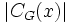$|C_G(x)|$ has order$h$ and let$C = C_G(x)$. Note that$x$ exists by the definition of$h$.
2. Let$y$ be an involution of$G$ that is not conjugate to$x$ and let$D = C_g(y)$: Note that$y$ exists because of the assumption that there are at least two conjugacy classes of involutions.
3. Let$y = y_1, y_2, \dots, y_t$ be distinct involutions of$D$, and define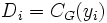$D_i = C_G(y_i)$. In particular,$D_1 = D$.
4.$t \le |D| \le h$:$t \le |D|$ because the$y_i$ are all distinct elements of$D$.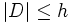$|D| \le h$ because$D$ is a centralizer of involution and by definition,$h$ is the maximum of the orders of such subgroups.
5. The number of distinct non-identity elements in$\bigcup_{i=1}^t D_i$ is less than$h^2$: Each$D_i$ has at most$h - 1$ non-identity elements, and there are$t$ of them, with$t \le h$. So, the total number of elements is less than$h^2$.
6. Suppose$x$ has a total of$m$ conjugates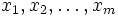$x_1, x_2, \dots, x_m$. Then, each$x_j$ is contained in the union$\bigcup_{i=1}^t D_i$: Any$x_j$ is conjugate to$x$. Since by assumption$x$ is not conjugate to$y$,$x_j$ is not conjugate to$y$. Thus, by fact (1), there exists an involution centralizing both$x_j$ and$y$. This involution lives in$C_G(y)$ so it is some$y_i$. Thus,$x_j \in C_G(y_i)$ for some$i$.
7.$m < h^2$: This follows from the previous two steps.
8.$|G| < h^3$: First, note that under the action of the group on itself by conjugation, the centralizer of$x$ is its stabilizer, so by fact (3), the coset space of$C_G(x)$ in$G$ is in bijection with the conjugacy class of$x$. Thus,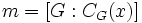$m = [G:C_G(x)]$. By fact (4) (Lagrange's theorem), we get$m = |G|/h$. Since$m < h^2$ by the previous step, we get$|G| < h^3$.